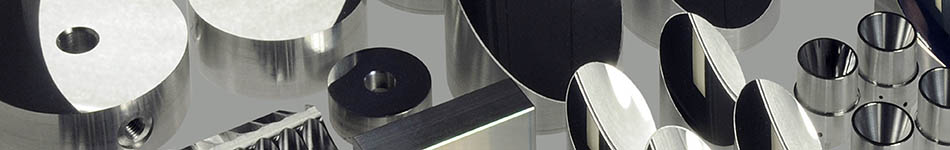//# Off-Axis Paraboloids Geometry Primer

## Paraboloids Defined

A parabola is defined by the conic section equation:

Ax^2 +Bxy + Cy^2 +Dx + Ey + F = 0, For a parabola B^2 = 4AC

Further reduction for a parabola with symmetry about the horizontal axis yields:

x = Ay^2 + By + C

The focus to vertex length (FVL) is defined as:

FVL = 1 / (4A)

The distance from the vertex to the center of the mirror is defined as:

CD = 2 FVL tan (a / 2)

'a' is the angle from the beam axis to the focus.

For concave paraboloid mirrors, light parallel to the beam axis will be reflected to the focus. This compares differently to concave spheric mirrors which reflect most of the light to a point. An off-axis paraboloid is a section of the parabola suited to provide unrestricted access to the mirror focal point.